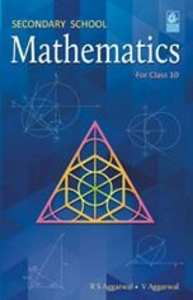Share

# R.S. Aggarwal solutions for Class 10 Mathematics chapter 13 - Constructions

## Secondary School Mathematics for Class 10 (for 2019 Examination)

#### R.S. Aggarwal Secondary School Mathematics Class 10 (for 2019 Examination)## Chapter 13: Constructions

#### Chapter 13: Constructions solutions [Page 0]

Draw a line segment AB of length 7 cm. Using ruler and compasses, find a point P on AB such that (AP)/(AB) = 3/5 .

Draw a line segment of length 7.6 cm and divide it in the ratio 5:8. Measure the two parts.

Construct a ΔPQR , in which PQ = 6 cm, QR = 7 cm and PR =- 8 cm. Then, construct another triangle whose sides are4/5 times the corresponding sides of ΔPQR .

Construct a triangle with sides 5 cm, 6 cm, and 7 cm and then another triangle whose sides are 7/5of the corresponding sides of the first triangle.

Construct a ΔABC with BC = 7 cm, ∠B = 60° and AB = 6 cm. Construct another triangle whose sides are 3/4 times the corresponding sides of ΔABC

Construct a ΔABC in which AB = 6 cm, ∠A = 30°  and ∠AB = 60° . Construct another ΔAB'C ' similar to ΔABC with base AB’ = 8 cm.

Construct a ΔABC in which BC = 8 cm, ∠B = 45° and ∠C = 60° . Construct another triangle similar to ΔABC such that its sides are 3/5of the corresponding sides of ΔABC .

To construct a triangle similar to ΔABC in which BC = 4.5 cm, ∠B = 45° and ∠C =60° , using a scale factor of 3/7, BC will be divided in the ratio
(a) 3 : 4 (b) 4 : 7 (c) 3 : 10 (d) 3 : 7

Construct an isosceles triangles whose base is 8 cm and altitude 4 cm and then another triangle whose sides are1/2 times the corresponding sides of the isosceles triangle.

Draw a right triangle in which the sides (other than hypotenuse) are of lengths 4 cm and 3cm. Then, construct another triangle whose sides are 5/3times the corresponding sides of the given triangle.

#### Chapter 13: Constructions solutions [Page 0]

Draw a circle of radius 3 cm. Form a point P, 7 cm away from the centre of the circle, draw two tangents to the circle. Also, measure the lengths of the tangents

Draw two tangents to a circle of radius 3.5 cm form a point P at a distance of 6.2 cm form its centre.

Draw a circle of radius 3.5 cm. Take two points A and B on one of its extended diameter, each at a distance of 5 cm from its center. Draw tangents to the circle from each of these points A and B.

Draw a circle with center O and radius 4 cm. Draw any diameter AB of this circle. Construct tangents to the circle at each of the two end points of the diameter AB.

Draw a circle with the help of a bangle. Take any point P outside the circle. Construct the pair of tangents form the point P to the circle

Draw a line segment AB of length 8 cm. Taking A as centre , draw a circle of radius 4 cm and taking B as centre , draw another circle of radius 3 cm. Construct tangents to each circle form the centre of the other circle.

Draw a circle of radius 4.2. Draw a pair of tangents to this circle inclined to each other at an angle of 45°

Write the steps of construction for drawing a pair of tangents to a circle of radius 3 cm , which are inclined to each other at an angle of 60° .

Draw a circle of radius 32 cm. Draw a tangent to the circle making an angle 30° with a line passing through the centre.

Construct a tangent to a circle of radius 4 cm form a point on the concentric circle of radius 6 cm and measure its length. Also, verify the measurement by actual calculation.

## Chapter 13: Constructions

#### R.S. Aggarwal Secondary School Mathematics Class 10 (for 2019 Examination)## R.S. Aggarwal solutions for Class 10 Mathematics chapter 13 - Constructions

R.S. Aggarwal solutions for Class 10 Maths chapter 13 (Constructions) include all questions with solution and detail explanation. This will clear students doubts about any question and improve application skills while preparing for board exams. The detailed, step-by-step solutions will help you understand the concepts better and clear your confusions, if any. Shaalaa.com has the CBSE Secondary School Mathematics for Class 10 (for 2019 Examination) solutions in a manner that help students grasp basic concepts better and faster.

Further, we at Shaalaa.com provide such solutions so that students can prepare for written exams. R.S. Aggarwal textbook solutions can be a core help for self-study and acts as a perfect self-help guidance for students.

Concepts covered in Class 10 Mathematics chapter 13 Constructions are Division of a Line Segment, Construction of Tangents to a Circle, Constructions Examples and Solutions.

Using R.S. Aggarwal Class 10 solutions Constructions exercise by students are an easy way to prepare for the exams, as they involve solutions arranged chapter-wise also page wise. The questions involved in R.S. Aggarwal Solutions are important questions that can be asked in the final exam. Maximum students of CBSE Class 10 prefer R.S. Aggarwal Textbook Solutions to score more in exam.

Get the free view of chapter 13 Constructions Class 10 extra questions for Maths and can use Shaalaa.com to keep it handy for your exam preparation

S